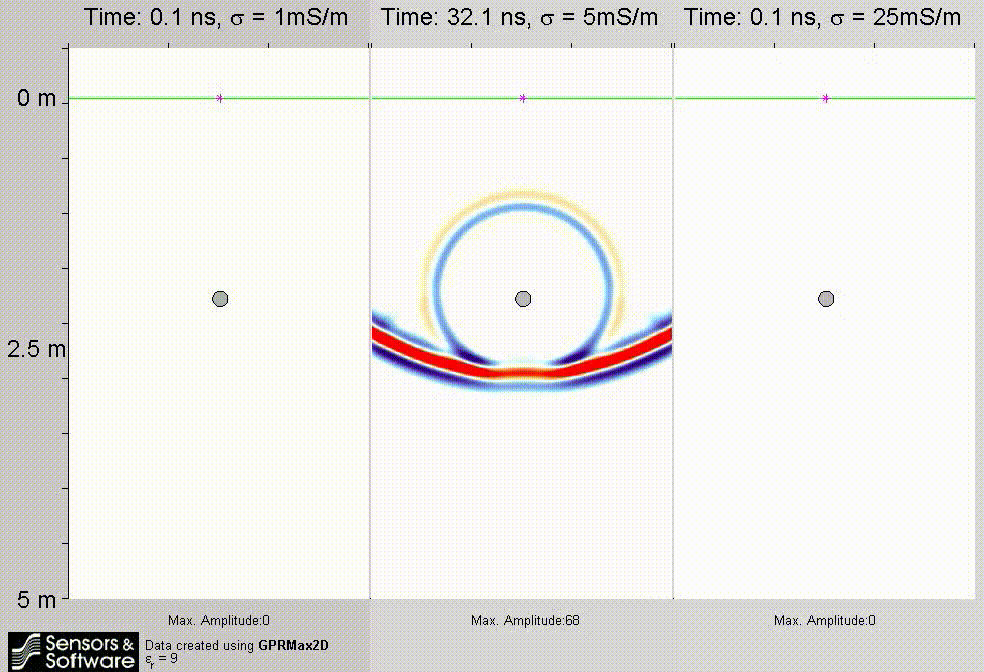TIPS: Estimating GPR PenetrationX

# TIPS: Estimating GPR Penetration

The very first question that someone usually asks about GPR is, “How deep can it see?”. Although we hear this question daily, we still do not have a quick, straight-forward answer for it. The shortest answer is, “It depends”. A better answer, but still not very specific is, “If you are working on fresh water, 1 to 40 meters, on the ground, 1 to 100 meters, on ice, even deeper”.

The reason that we cannot answer this question simply is because the depth of penetration of GPR depends on many factors, including the GPR antenna frequency, the GPR transmitter power, the number of stacks, scattering losses in heterogeneous materials and the level of background radio frequency noise. However, the biggest factor is the electrical properties of the material being scanned, specifically the electrical conductivity of that material (Figure 1).

Most people have heard that clay soils are “bad” for GPR penetration. The underlying reason that clay-rich soils are not good for deep penetration with GPR is because clay has high electrical conductivity. Other common materials with high electrical conductivity are silty soils, sea water and, of course, metal. One of the first lessons that GPR operators learn is not to expect to see through metal.Figure 1
The electrical conductivity of the material being scanned controls the attenuation of the signal. Lower electrical conductivity
(left) allows GPR signals to travel deeper into the medium than high electrical conductivity (right).

### Estimating GPR Penetration in the Ground

Occasionally, we have a customer that has a measurement of the electrical conductivity of an area they are interested in scanning with GPR. This information is often from another geophysical survey conducted in the area, such as an electrical resistivity survey or an electromagnetic (EM) survey. With this information, the depth of penetration that would be achieved with GPR in meters can be estimated by the expression:

Depth (m) = 40/σ
where, σ is electrical conductivity expressed in millisiemens per meter (mS/m)

If the measurement is resistivity in ohm-meters, the formula becomes:

Depth (m) = ρ/25

where, ρ is resistivity expressed in ohm-meters (ohm-m)

### Estimating GPR Penetration in Fresh Water

If you are interested in using GPR on fresh water, there is also a formula, related to the ones above, for estimating the depth of penetration when using GPR. It requires a property of water, commonly measured by hydrologists, called the total dissolved solids or TDS. If you have a measurement of TDS of the water you want to scan with GPR, use this expression to estimate the depth of GPR penetration in the water:

Depth (m) = 1850/TDS

where, TDS is measured in milligrams per liter (mg/l) or parts per million (ppm)

The table below summarizes these formulas using typical values for electrical conductivity and resistivity values for the various materials. Remember: the formulas provide a best-case estimate of the depth of penetration; additional factors, including antenna frequency, transmitter power and receiver sensitivity as mentioned above, also need to be considered. As well, rarely are GPR surveys conducted through a pure material; most often the subsurface is a heterogeneous mixture of different materials resulting in signal scattering which also reduces penetration.

Material Electrical Conductivity (mS/m) Electrical Resistivity (ohm-m) Total Dissolved Solids (ppm) Depth of Penetration (m)
Air 0 0
Ice, Snow, 0.1 10,000 400+
Granite, Marble 0.4 2500 100
Dry sand, Limestone 1 1000 40
Wet sand, gravel 2 500 20
Silt 20 50 2
Clay, concrete 50 20 1
Fresh water (low TDS) 45 40
Fresh water (high TDS) 3700 0.5
Sea water 40,000 0.01

Check out Frequently asked questions: GPR FAQs

﻿

﻿